Next: Equation of motion under Up: Solving Equation of Motion Previous: Solving Equation of Motion

### Equation of motion under DRIFT EXTERNAL command

The present version of CAIN accepts a constant external field only. The covariant form of the equation of motion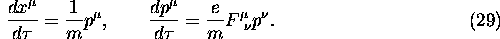where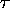is the proper time and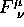the electromagnetic field tensor, can be solved exactly when the field is constant. The eigenvalues of the matrix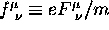is given by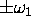and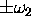, where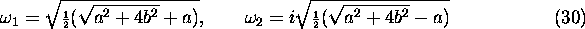with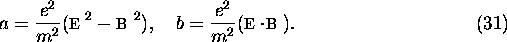Then, the solution is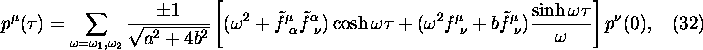where the upper (lower) sign applies to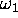(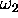) and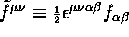with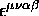being the antisymmetric tensor of rank 4.

The classical spin motion of electrons is given by the Thomas-BMT equation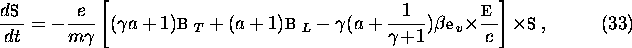where a is the coefficient of anomalous magnetic moment  and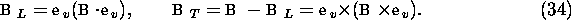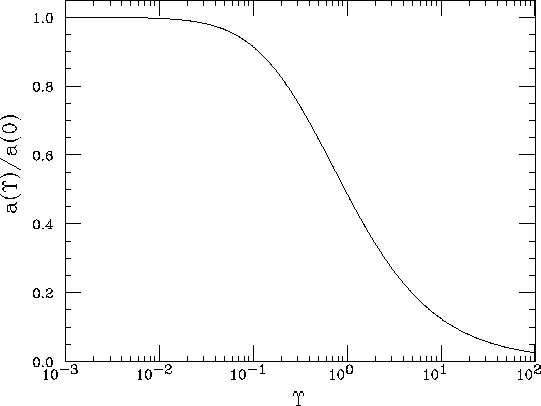Figure 1: Field dependence of the anomalous magnetic moment of electron

When the field is very strong, a is different from the well-known value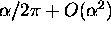but is a function of the field strength characterized the parameter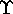.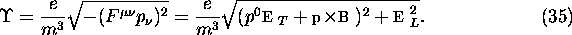The functional form of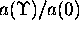is shown in Fig.1. Simple polynomial approximations are used in CAIN.

Toshiaki Tauchi
Thu Dec 3 17:27:26 JST 1998